When you get a container of tabletop rpg dice you will notice that the dice come in many shapes.  To players unfamiliar with tabletop rpg’s some dice can be confusing to read. But worry not for this article will explain how to read and understand all of the tabletop rpg dice!

I will be giving you an overview of all the dice that are used in tabletop RPG’s. Although Dungeons & Dragons and similar games use all the dice, not every system does. Keep this in mind if you are looking to buy dice for the first time.

## First Some Terminology

Most of the time whether said or in rules dice are referred to as “D” and a number afterwards. D stands for dice and the number stands for the number of sides on that dice. For example a D10 refers to a 10 sided die.

The other commonly used diced term is percentile dice. This is actually two dice hence the term percentile dice not percentile die. This will be explained further down.

## The Reliable D20

The 20 sided die is the most common dice used in Dungeons & Dragons. Besides being the most common dice, the system Dungeons & Dragons is part of is called the D 20 system.

One thing you need to look out for is that there are two different D 20s in circulation. Magic the Gathering uses a countdown D 20 while D 20 systems do not. Be careful not to mix the two up when buying dice. The way you tell a difference is that countdown die when looking at the 20 will have decreasing numbers surrounding it. The die is basically split in half between high numbers and low numbers. A tabletop D 20 has the numbers are scattered around the die so that there is an even mix.

Just because a countdown d20 is not used for rolling does not make them useless. I use them all the time to keep track of spell slots or daily uses.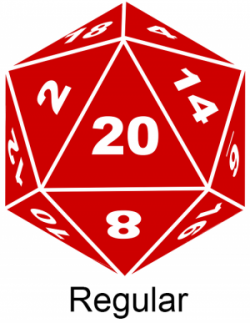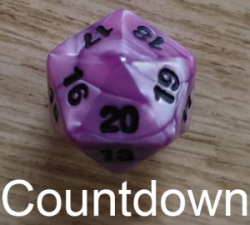## The Relatively Unknown D12

The 12 sided dice has the distinction of not being used regularly. Most systems that have a D12 only use it for select weapons.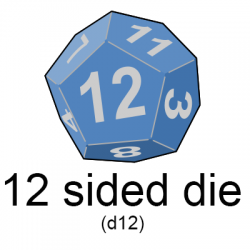## The D10

When you buy a set of dice upon first glance it looks like you get two D10’s. This is because of these two dice make up the percentile dice. One thing that is great about this is that if you need it, negate the zero on the tens percentile die and you effectively get two D10’s. One thing to keep in mind is that the 0 on a D10 represents a 10 not 0.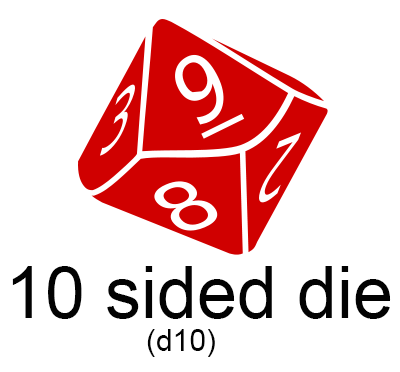## The Challenging Percentile Dice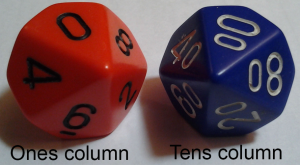These are always the most confusing tabletop rpg dice. As described above percentile dice are the two D10’s. One is a regular the other has a zero after all the numbers. Because of how these work new players often find that these dice the most confusing to read.

The way to read it is to consider the D10 with just one number as the ones column of a number. For example if you’re the role 5 on that die you have a 5 in the ones column. The dice with two digits is the tens column. For example if you were to roll a 20 on that die the number will be 20 not a 2 because the 2 in in the tens column. The trick is that to get the number rolled you add the two dice together. For example add the 20 on the two digit die with the 5 on the single digit die to get 25.

Rolling 100 or rolling 1-9 get a little more complicated. The 00 when added with a 1-9 on the single die gives you the number in the single digits, for example if you roll 00 and a 4 the number you get is 4. This changes however if you roll a 00 and then of 0 on the single digit die. When this is done you get 100.

Remember 000 = 100

One trick I tell players is that if you roll a 00 and a 1-9 on the single digit die move the 00 from sight and only read the single digit die.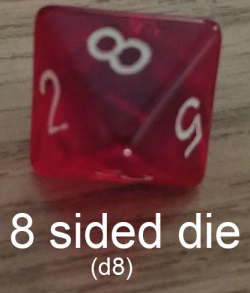## D8

The eight sided die is most commonly confuse for the D10 and vice versa. This is because they both have that diamond shape. The trick to keep them separate is that not all sides on the face of the D10 are straight while on the D8 the face makes a triangle with three straight sides.

## The Standard D6

This die needs no introduction, being the most common die in almost every board game.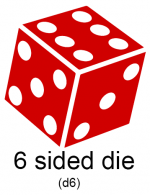## The Two Styles of D4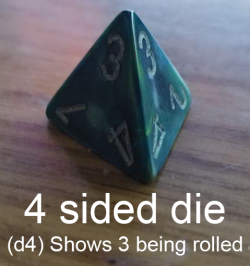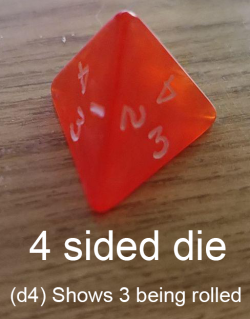This dice is sometimes referred to as a pyramid and is one of the most interesting looking dice in a set. This is because unlike the rest of the dice they have multiple numbers on each face. The D4, unlike the rest of the dice used in tabletop rps’s,  show the number in two different ways. The reason this die is like this is because there is always more than one face showing therefore when you roll the die each face shows the same number the matter which face you’re looking at.

The first one has a number on each angle of the triangle face. When you roll this dice the number you roll is at the peak of the dice or the top of the pyramid. For example if you to roll a 3 there will be the number 3 on each face of the die at the peak where the points converge.

The other way D4 is can show the number is at the bottom. This die has their number centered along one of the sides the face instead of at the angles. Unlike the other version when you roll, the number you get is at the bottom of the die not the peak.